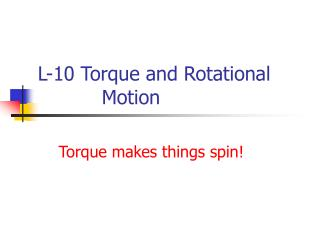DownloadDownload PresentationL-10 Torque and Rotational Motion

# L-10 Torque and Rotational Motion

Télécharger la présentation## L-10 Torque and Rotational Motion

- - - - - - - - - - - - - - - - - - - - - - - - - - - E N D - - - - - - - - - - - - - - - - - - - - - - - - - - -
##### Presentation Transcript

1. L-10 Torque and Rotational Motion Torque makes things spin!

2. What makes something rotate in the first place? TORQUE How do I apply a force to make the rod rotate about the axel? Not just anywhere! AXEL

3. TORQUE • To make an object rotate, a force must be applied in the right place. • the combination of force and point of application is called TORQUE lever arm, L Axle Force, F

4. Torque = force times lever arm Torque = F  L

5. Torque example What is the torque on a bolt applied with a wrench that has a lever arm of 30 cm with a force of 10 N? F Torque = F  L = 30 N  0.30 m = 9 N m L For the same force, you get more torque with a bigger wrench  the job is easier!

6. Net Force = 0 , Net Torque ≠ 0 10 N 10 N • > The net force = 0, since the forces are applied in • opposite directions so it will not accelerate. • > However, together these forces will make the rod • rotate in the clockwise direction.

7. Net torque = 0, net force ≠ 0 The rod will accelerate upward under these two forces, but will not rotate.

8. Balancing torques 20 N 10 N 0.5 m 1 m Left torque = 10 N x 1 m = 10 n m Right torque = 20 N x 0.5 m = 10 N m

9. Equilibrium • To ensure that an object does not accelerate or rotate two conditions must be met: •  net force = 0 •  net torque = 0 • this results in the practical 4-1 “ladder rule”

10. When is an object stable? • If you can tip it over a bit and it doesn’t fall • The object may wobble a bit but it eventually stops and settles down to its upright position.

11. Why things fall over • Every object has a special point called the center of gravity (CG). The CG is usually right smack in the center of the object. • if the center of gravity is supported, the object will not fall over. • You generally want a running back with a low CG then it’s harder to knock him down. • The lower the CG the more stable an object is. stable  not easy to knock over!

12. CG Condition for stability If the CG is above the edge, the object will not fall

13. CG CG when does it fall over? If the vertical line extending down from the CG is inside the edge the object will return to its upright position  the torque due to gravity brings it back. STABLE NOT STABLE

14. Stable and Unstable stable unstable torque due to gravity pulls object back torque due to gravity pulls object down

15. Stable structures Structures are wider at their base to lower their center of gravity

16. Playing with your blocks CG If the center of gravity is supported, the blocks do not fall over

17. Object with low CG 300 lb fullback who is 4 ft, 10 inches tall and runs a 4-40 Stay low to the ground!

18. High Profile Vehicles wind As more and more stuff is loaded into a semi, its center of gravity moves upward. It could be susceptible to tipping over.

19. The shape of an object determines how easy or hard it is to spin Hinge For objects of the same mass, the longer one is tougher to spin  takes more torque

20. It matters where the hinge is The stick with the hinge at the end takes 4 times more torque to get it spinning than the stick with the hinge in the center.

21. Rotational Inertia (moment of inertia) • Rotational inertia is a parameter that is used to quantify how much torque it takes to get a particular object rotating • it depends not only on the mass of the object, but where the mass is relative to the hinge or axis of rotation • the rotational inertia is bigger, if more mass is located farther from the axis.

22. How fast does it spin? • For spinning or rotational motion, the rotational inertia of an object plays the same role as ordinary mass for simple motion • For a given amount of torque applied to an object, its rotational inertia determines its rotational acceleration  the smaller the rotational inertia, the bigger the rotational acceleration

23. Same torque, different rotational inertia Big rotational inertia Small rotational inertia spins fast spins slow

24. rotational inertia - examples Suppose we have a rod of mass 2 kg and length 1 meter with the axis through the center Its moment of inertia is 2 units Imagine now that we take the same rod and stretch it out to 2 meters; its mass is, of course, the same. Its moment of inertia is 4 units

25. rotational inertia examples Rods of equal mass and length axes through center Rotational inertia of 1 unit axes through end Rotational inertia of 4 units